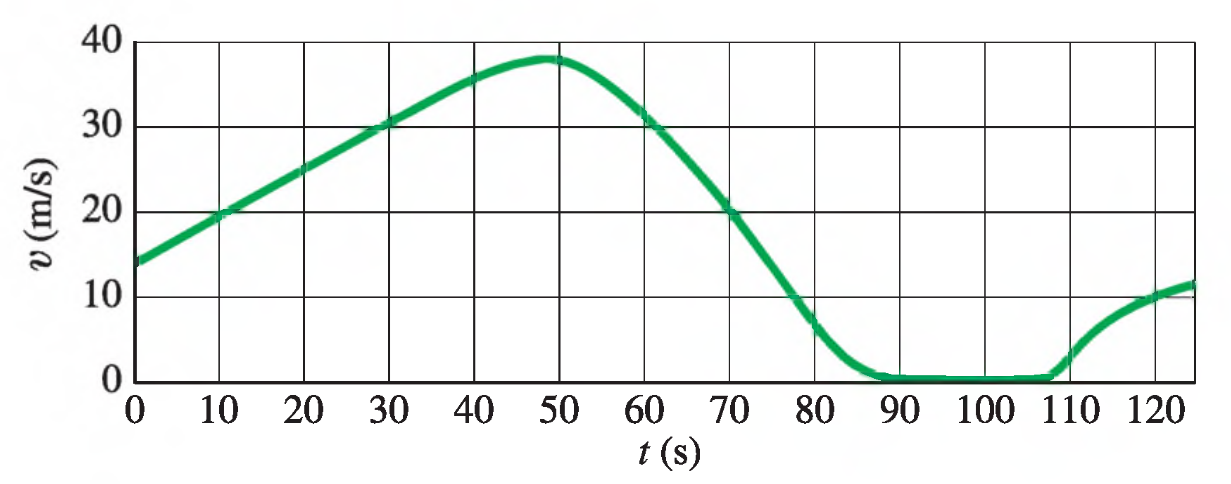Physics Practice Problems Velocity & Acceleration Graphs Practice Problems Solution: The figure shows the velocity of a train as a func...

# Solution: The figure shows the velocity of a train as a function of time. (a) At what time was its velocity greatest?(b) During what periods, if any, was the velocity constant?(c) During what periods, if any, was the acceleration constant?(d) When was the magnitude of the acceleration greatest?

###### Problem

The figure shows the velocity of a train as a function of time.

(a) At what time was its velocity greatest?

(b) During what periods, if any, was the velocity constant?

(c) During what periods, if any, was the acceleration constant?

(d) When was the magnitude of the acceleration greatest?###### Solution

We're looking at a velocity graph to determine some things about the velocity and acceleration of at train.

Anytime we're given a position, velocity, or acceleration graph to work with, a PVA diagram like the one below can help remind you of the relationships between the three functions.

$\mathbit{P}\begin{array}{c}{\mathbf{←}}\\ {\mathbf{\to }}\end{array}\underset{{\mathbit{s}}{\mathbit{l}}{\mathbit{o}}{\mathbit{p}}{\mathbit{e}}}{\overset{{\mathbit{a}}{\mathbit{r}}{\mathbit{e}}{\mathbit{a}}}{\mathbit{V}}}\begin{array}{c}{\mathbf{←}}\\ {\mathbf{\to }}\end{array}\mathbit{A}$

Moving left to right, we take the slope of the graph—the slope of a position graph is the velocity, and the slope of a velocity graph is the acceleration.View Complete Written SolutionVelocity & Acceleration Graphs

Velocity & Acceleration Graphs

#### Q. The figure shows the position vs. time graph for two bicycles, A and B.Is there any instant at which the two bicycles have the same velocity?Which bic...

Solved • Mon Oct 29 2018 19:00:17 GMT-0400 (EDT)

Velocity & Acceleration Graphs

#### Q. The graph in the figure describes the acceleration as a function of time for a stone rolling down a hill starting from rest. (a) Find the change in th...

Solved • Mon Oct 29 2018 18:50:20 GMT-0400 (EDT)

Velocity & Acceleration Graphs

#### Q. High-speed motion pictures ( 3500 ) of a jumping, 210 mu { m{g}} flea yielded the data used to plot the graph given in the figure . (See "The Flying L...

Solved • Mon Oct 29 2018 18:49:58 GMT-0400 (EDT)

Velocity & Acceleration Graphs

#### Q. A cat walks in a straight line, which we shall call the x-axis with the positive direction to the right. As an observant physicist, you make measureme...

Solved • Mon Oct 29 2018 18:49:57 GMT-0400 (EDT)